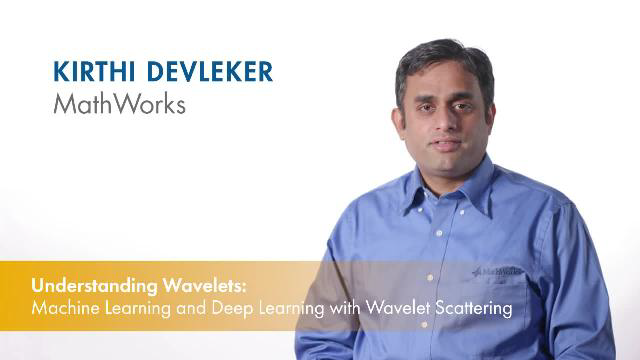# Get Started with Wavelet Toolbox

Analyze and synthesize signals and images using wavelets

Wavelet Toolbox™ provides apps and functions for analyzing and synthesizing signals and images. You can detect events like anomalies, change points, and transients, and denoise and compress data. Wavelet and other multiscale techniques can be used to analyze data at different time and frequency resolutions and to decompose signals and images into their various components. You can use wavelet techniques to reduce dimensionality and extract discriminating features from signals and images to train machine and deep learning models.

With Wavelet Toolbox you can interactively denoise signals, perform multiresolution and wavelet analysis, and generate MATLAB® code. The toolbox includes algorithms for continuous and discrete wavelet analysis, wavelet packet analysis, multiresolution analysis, wavelet scattering, and other multiscale analysis.

Many toolbox functions support C/C++ and CUDA® code generation for desktop prototyping and embedded system deployment.

## VideosUnderstanding Wavelets, Part 1: What Are Wavelets
Explore the fundamental concepts of wavelet transforms in this introductory MATLAB Tech Talk. This video covers what wavelets are and how you can use them to explore your data in MATLAB. The video focuses on two important wavelet transform concepts: scaling and shifting. The concepts can be applied to 2-D data such as images.Understanding Wavelets, Part 2: Types of Wavelet Transforms
Explore the workings of wavelet transforms in detail. You will learn more about the continuous wavelet transforms and the discrete wavelet transform. You will also learn important applications of using wavelet transforms with MATLAB.Understand Wavelets, Part 3: An Example Application of the Discrete Wavelet Transform
Learn how to use to wavelets to denoise a signal while preserving its sharp features in this MATLAB Tech Talk. This video outlines the steps involved in denoising a signal with the discrete wavelet transform using MATLAB. Learn how this denoising technique compares with other denoising techniques.Understanding Wavelets, Part 4: An Example Application of the Continuous Wavelet Transform
Explore a practical application of using continuous wavelet transforms in this MATLAB Tech Talk. Get an overview of how to use MATLAB to obtain a sharper time-frequency analysis of a signal with the continuous wavelet transform. This video uses an example seismic signal to highlight the frequency localization capabilities of the continuous wavelet transform.Understanding Wavelets, Part 5: Machine Learning and Deep Learning with Wavelet Scattering
Wavelet scattering networks help you automatically obtain low-variance features from signals and images for use in machine learning and deep learning applications. In this MATLAB Tech Talk, learn about the wavelet scattering transform and how it can be used as an automatic robust feature extractor for classification.

## SupportGet trial now